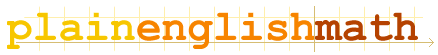You are here: MathematicsFundamentals → The Greek Alphabet

# The Greek Alphabet

Using letters of the alphabet to stand in place of values in equations does pose one small problem - there are only 26 letters in the Latin alphabet (which the English language uses, and this website is written in), and at times, re-using the same letter to stand for different things in different equations can get confusing.

As a consequence, mathematicians often mix Greek letters into equations as well.

Here are the upper and lowercase letters of the Greek alphabet, together with their names:

 Α α alpha Β β beta Γ γ gamma Δ δ delta Ε ε epsilon Ζ ζ zeta Η η eta Θ θ theta Ι ι iota Κ κ kappa Λ λ lambda Μ μ mu Ν ν nu Ξ ξ xi Ο ο omicron Π π pi Uppercase Π usually represents a product series, while lowercase π represents the ratio 'pi' Ρ ρ rho Σ σ sigma Uppercase usually represents a sum series. Τ τ tau Υ υ upsilon Φ φ phi Χ χ chi Pronounced: "kye" Ψ ψ psi Ω ω omega